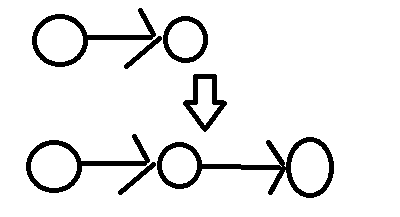bzoj3669 [Noi2014]魔法森林

3669: [Noi2014]魔法森林

Time Limit: 30 Sec  Memory Limit: 512 MB
Submit: 3287  Solved: 2100
[Submit][Status][Discuss]

【输入样例1】
4 5
1 2 19 1
2 3 8 12
2 4 12 15
1 3 17 8
3 4 1 17
【输入样例2】
3 1
1 2 1 1

【输出样例1】
32
【样例说明1】

【输出样例2】
-1
【样例说明2】

HINT

2<=n<=50,000

0<=m<=100,000

1<=ai ,bi<=50,000

两个量ai,bi影响答案，一个非常常见的做法就是对每条边以ai为关键字从小到大排序，这样从1到m枚举每条边，那么当前枚举到的ai就是最大的a了. 只需要使得路径上最大的b最小就好了.

这道题的目的是使得1,n连通，并且1,n路径上最大的a加上最大的b的和最小. 当前已经知道了最大的a是多少，剩下的就是使得1,n连通并且让最大的b最小.

维护无向图的连通性可以用并查集.

考虑第i条边的时候，如果这条边连接的两个点没有连通，直接加上这条边就好了.

如果连通了，则找到这两个点链上的最大的b,如果第i条边的b比这个最大的b要小，那么第i条边肯定要优，删掉那条拥有最大的b的边，加入第i条边.

这个删边的方法是最小生成树问题上一个比较常用的方法，具体可见：传送门.

怎么支持加边删边？ LCT！

LCT只能维护点权，这道题要维护边权怎么办啊？例如这张图，上面的表示原来的边(x,y). 在x,y中间插入一个点k. k点的点权就是(x,y)的b. 每次加边先加(x,k)，再加(k,y),删边是类似的.

需要注意的是：加入的点序号不能与原splay中的点重复，也就是说：如果这个点属于第i条边，那么这个点的编号就是i+n. 删边和加边的时候要注意!

Get到了LCT的新套路，想到对ai排序后就没往连通性那方面去想了，经验不足啊.  其实遇到这类2个因素影响答案的问题，就应该往两个方面去想：1.对其中一个进行排序，消除影响,例如：传送门.  2.变成二元组放在平面直角坐标系上，看看有没有什么性质能够对其进行优化,例如：bzoj1597.

#include <cstdio>
#include <cstring>
#include <iostream>
#include <algorithm>

using namespace std;

const int maxn = 200010,inf = 0x7ffffff;

int n,m,f[maxn],val[maxn],ans = inf,sta[maxn];
int fa[maxn],son[maxn],maxx[maxn],rev[maxn];

struct node
{
int x,y,a,b;
}e[maxn];

bool cmp(node p,node q)
{
if (p.a == q.a)
return p.b < q.b;
return p.a < q.a;
}

bool is_root(int x)
{
return son[fa[x]] != x && son[fa[x]] != x;
}

bool get(int x)
{
return son[fa[x]] == x;
}

int find(int x)
{
if (x == f[x])
return x;
return f[x] = find(f[x]);
}

int pushdown(int x)
{
if (rev[x])
{
rev[son[x]] ^= 1;
rev[son[x]] ^= 1;
rev[x] = 0;
swap(son[x],son[x]);
}
}

void pushup(int x)
{
maxx[x] = x;
if (son[x])
if (val[maxx[son[x]]] > val[maxx[x]])
maxx[x] = maxx[son[x]];
if (son[x])
if (val[maxx[son[x]]] > val[maxx[x]])
maxx[x] = maxx[son[x]];
}

void turn(int x)
{
int y = fa[x];
int z = fa[y];
int temp = get(x);
if (!is_root(y))
son[z][son[z] == y] = x;
fa[x] = z;
son[y][temp] = son[x][temp ^ 1];
fa[son[y][temp]] = y;
son[x][temp ^ 1] = y;
fa[y] = x;
pushup(y);
pushup(x);
}

void splay(int x)
{
int top = 0;
sta[++top] = x;
for (int y = x; !is_root(y); y = fa[y])
sta[++top] = fa[y];
for (int i = top; i >= 1; i--)
pushdown(sta[i]);
int temp;
for (; !is_root(x); turn(x))
{
if (!is_root(temp = fa[x]))
{
if (get(x) == get(temp))
turn(temp);
else
turn(x);
}
}
}

void Access(int x)
{
int p = 0;
for (; x; p = x,x = fa[x])
{
splay(x);
son[x] = p;
pushup(x);  //注意pushup
}
}

void Reverse(int x)
{
Access(x);
splay(x);
rev[x] ^= 1;
}

void Cut(int x,int y)
{
Reverse(x);
Access(y);
splay(y);
son[y] = fa[x] = 0;
}

{
Reverse(x);
fa[x] = y;
splay(x);
}

int query(int x,int y)
{
Reverse(x);
Access(y);
splay(y);
return maxx[y];
}

int main()
{
scanf("%d%d",&n,&m);
for (int i = 1; i <= m; i++)
scanf("%d%d%d%d",&e[i].x,&e[i].y,&e[i].a,&e[i].b);
sort(e + 1,e + 1 + m,cmp);
for (int i = 1; i <= n; i++)
f[i] = i;
for (int i = 1; i <= m; i++)
val[i + n] = e[i].b;
for (int i = 1; i <= m; i++)
{
int x = e[i].x,y = e[i].y;
int fx = find(x),fy = find(y);
if (fx != fy)
{
f[fx] = fy;
}
else
{
int k = query(x,y);
if (val[k] > e[i].b)
{
Cut(e[k - n].x,k);
Cut(k,e[k - n].y);
}
}
if (find(1) == find(n))
ans = min(ans,e[i].a + val[query(1,n)]);
}
if (ans == inf)
printf("%d\n",-1);
else
printf("%d\n",ans);

return 0;
}

posted @ 2018-03-08 22:01  zbtrs  阅读(159)  评论(0编辑  收藏  举报